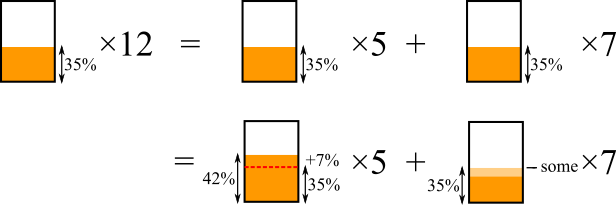#### You may also like### Percentage Unchanged

If the base of a rectangle is increased by 10% and the area is unchanged, by what percentage is the width decreased by ?### Circle Packing

Equal circles can be arranged so that each circle touches four or six others. What percentage of the plane is covered by circles in each packing pattern? ...### Round and Round

Prove that the shaded area of the semicircle is equal to the area of the inner circle.

# Pineapple Juice

##### Age 14 to 16 Short Challenge Level:

Answer: $30\%$

Using ratio and parts
$450$ and $630$ are in the ratio $45:63 = 5:7$
$$\begin{array} \text{5 parts 42%} \\ \text{7 parts ?%} \end{array} \bigg\} \text{12 parts 35%}$$

Working backwards from the new juice,$\qquad\qquad\qquad\qquad\qquad\qquad\qquad\qquad+7\%\times5$ balances with $-\text{some}\times7$
$\qquad\qquad\qquad\qquad\qquad\qquad\qquad\qquad\Rightarrow 35\%=\text{some}\times7$
$\qquad\qquad\qquad\qquad\qquad\qquad\qquad\qquad\Rightarrow \text{some}=5\%$

$35\%-5\%=30\%\therefore$ the orange drink was 30% juice.

Finding the amounts of juice
$450 + 630 = 1080$

$42\%$ of $450 =42\%$ of $100\times4.5=42\times4.5 = 189$

$35\%$ of $1080= 35\%$ of $1000 + 35\%$ of $80$, which is $350 + 24 + 4 = 378$

$378-189=189 \therefore 189$ litres of juice comes from $630$ litres orange drink

$\dfrac{189}{630}=\dfrac{63}{210}=\dfrac{21}{70}=\dfrac{3}{10}=30\%$

Using ratio and total juice
$450$ and $630$ are in the ratio $45:63 = 5:7$
$$\begin{array} \text{5 parts 42%} \\ \text{7 parts ?%} \end{array} \bigg\} \text{12 parts 35%}$$

Since we are working in 'parts' and not specific units, we can simply multiply by 42 and 35, instead of finding 42% and 35%, because 42% and 35% are in the same ratio as 42 and 35.

$42\times5 + ?\times7 = 35\times12$
$\Rightarrow7\times6\times5 + 7\times? = 7\times5\times12$
$\Rightarrow7\times(6\times5 + ?) = 7\times5\times12$
$\Rightarrow6\times5 + ? = 5\times12$
$\Rightarrow30 + ? = 60$
$\Rightarrow? = 30$

Using fractions
450 and 630 are in the ratio 45:63 = 5:7, so the mixture is $\frac5{12}$ pineapple drink and $\frac7{12}$ orange drink.

So we know that 42% of $\frac5{12}$ of the mixture is juice and that 35% of the whole mixture is juice. Let $x$ be the fraction of the orange drink that is juice. Then:
\begin{align} \frac{42}{100}\times\frac5{12}\hspace{6mm}+\hspace{5mm}x\times\frac{7}{12}&=\hspace{3mm}\frac{35}{100}&\\ \Rightarrow \frac{6\times7}{20\times5}\times\frac{5}{6\times2}\hspace{1mm}+\hspace{5mm}x\times\frac7{12}&=\frac{7\times5}{20\times5}\\ \Rightarrow \frac7{20\times2}\hspace{8mm}+\hspace{5mm}x\times\frac7{12}&=\hspace{3mm}\frac{7}{20}\\ \Rightarrow x\times\frac7{12}&=\frac{7\times2}{20\times2}-\frac7{20\times2}\\ \Rightarrow x\times\frac7{6\times2}&=\frac{7}{20\times2}\\ \Rightarrow x\times\frac16&=\frac1{20}\\ \Rightarrow x&=\frac6{20}\\ \Rightarrow x&=\frac3{10} \end{align} Which is the same as 30%.

You can find more short problems, arranged by curriculum topic, in our short problems collection.